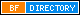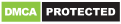# How to calculate Returns on your Fixed Deposits

A fixed deposit is a popular and frequently opted for investment scheme in India. The reason behind its popularity are benefits such as guaranteed returns as well as the provision of a risk-free nature. FD rates being quite higher than savings account rates is also another reason behind the ensuing popularity of the scheme. But how exactly do these interest rates apply on your deposit amount to give you returns? Scroll down to understand the process in detail.

## How to calculate Returns on your Fixed Deposits

### Fixed deposit calculator

An FD calculator assists the person who is investing the money to have prior and proper knowledge of the returns they are going to gain from investing in the scheme. Depending on the choice of the investor, the interest on FD is either paid out on a regular interval basis or at the maturity of the principal amount. The investor will receive more returns if the tenure is long and the deposit is a significantly large amount. The formula of compounding interest is applied to assure return on interest. Online fixed deposit calculators are almost always correct in giving an approximate estimate of the expected returns. This tool is free of cost, easy to use and provides the result almost instantly.

### Formula to calculate fixed deposit

Returns against a fixed deposit account are determined by three primary factors, namely, the principal amount that you are investing, the tenure for which you wish to invest and the interest rate of the bank or the Non-banking financial company (NBFC). Out of this, the interest rate is predetermined and set by the bank or NBFC and the rest two factors are flexible. Senior citizens are given a higher rate of interest on FD which is called the ’preferential interest rate’. The age to be considered a senior citizen is generally between 55 to 60 years. However, this varies from one bank to another.

### Simple interest method to calculate returns on your FD

This finds out the interest earned on the principal amount at the particular rate and for the particular time period. The formula for calculating this is: Simple Interest = ( P * R * T ) / 100; where P stands for the principal amount that you have invested. R stands for the rate of interest at which you’ve invested and T for the tenure.

### Compound interest method to calculate returns on FD

This the interest that is earned on the principal amount invested and the interest that is earned from that. The interest is increased to the number of years for which the interest is going to be compounded. The formula for calculating this is: A = P (1+r/n) ^ (n * t); where A refers to the Maturity amount, P stands for the principal amount, r for the rate of interest , n stands for number compounding per year and t for the tenure.

### Conclusion

The question regarding which method is more profitable may arise. Most banks use the compounding method while many banks use the simple interest method, too. It is, however, necessary for you to decide which is more suitable for you and make a choice accordingly.This site uses Akismet to reduce spam. Learn how your comment data is processed.blogging tips Follow my blog with Bloglovin